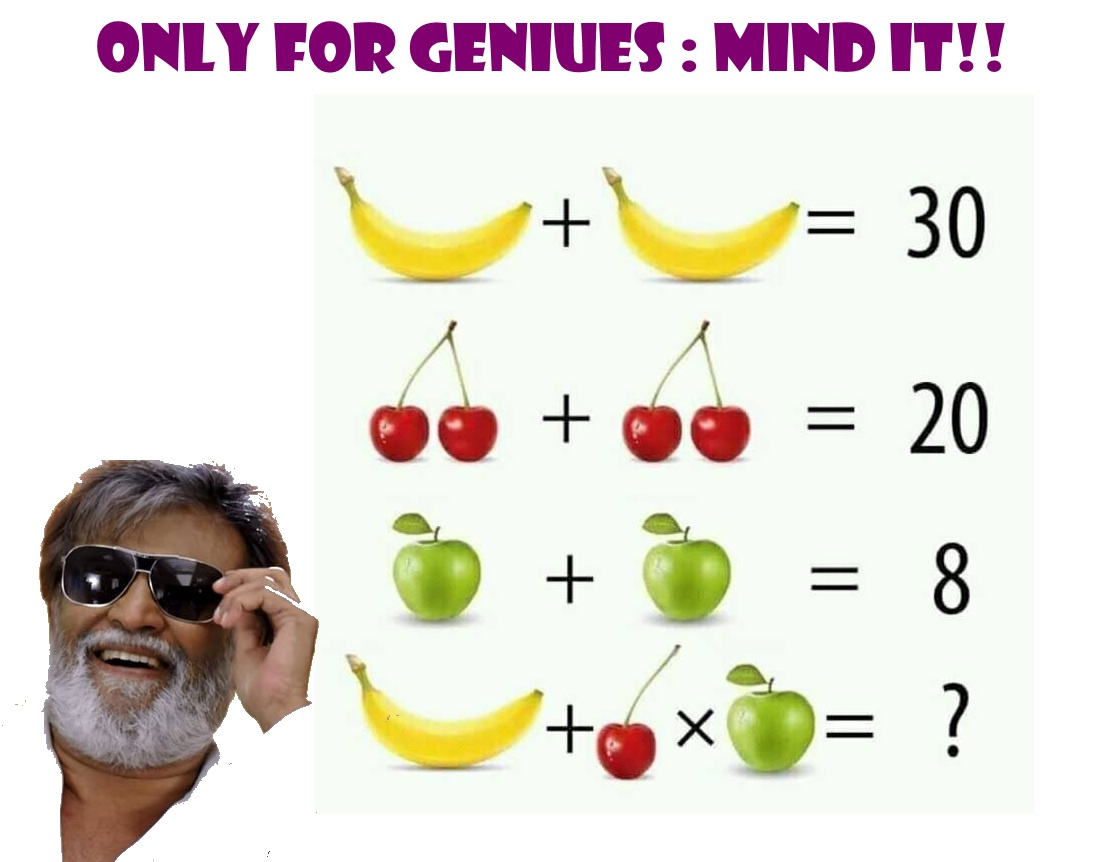This post may contain affiliate links. This means if you click on the link and purchase the item, We will receive an affiliate commission at no extra cost to you. See Our Affiliate Policy for more info.

# Banana + Cherry x Apple =?? COOL Math Puzzles Only For Geniuses!

6
5727

Hello Puzzle Lovers, Here an interesting puzzle you to solve. In this Fruit Puzzle, You have to find the value of the 4th equation. Three equation is given above. You have to find the value of Banana, Cherry and Apple. And then Solve the Last Equation.

It’s not that simple as you think quite it is? Observe the Puzzle picture carefully First and then solve.

## Only For Geniuses : Cool Math Puzzles

Solve this puzzles and let’s see you are genius or not? If your answer is not Correct. Don’t worry Solution is given Below.

# Banana + Cherry  x  Apple = ??  Solve it Geniuses!!Equation (1)

Banana + Banana = 30            ( Banana= b)

b + b = 30

b = 15

Banana = 15

Equation (2)

2 Cherry + 2 Cherry = 20        (Cherry = c)

2c + 2c = 20

4c = 20

c = 5

Cherry = 5

Equation (3)

Apple + Apple = 8                   (Apple = a)

a + a= 8

a = 4

Apple= 4

Equation (4)

Banana + Cherry  x  Apple = ?

15 + 5 x 4 = ?                                  (First Multiplication then Addition)

15 + 20 = 35

Search Items:-

Apple Banana Puzzles, Fruit Math Puzzles, Tricky Maths Puzzles, Fruit Puzzle with Answer, Math Puzzles with Answers, Cool Math Puzzles, Cool Math Riddles, Cool Brain Games, Only For Genius, Only For Geniuses Math Puzzles, Math Puzzles with Answer, Puzzles For Facebook, Puzzles For Whats App, Brain Booster Riddles, Brain Games, Tricky Mind Riddles, Puzzles and Riddles, Math Problems, Fruit Brainteaser Puzzle Image, Brainteaser Puzzles,Apple Cherry Banana Fruit Puzzle, Pics Story

1.Ronda

Simplifying
5 + 1 * 10 = 60

2.Pachuko

Which answer is right 35 or 80

•Kumar

I think 35 😉

3.Shafu

39👍